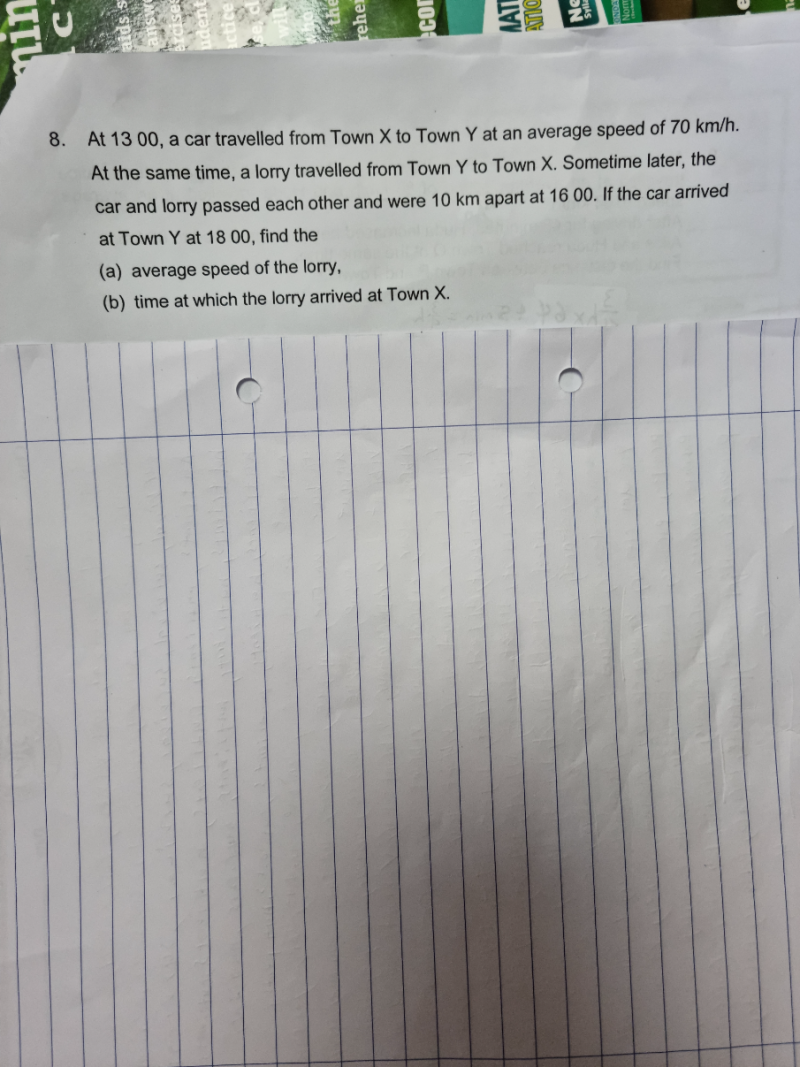# QuestionThank you.

Car speed = 70km/h

Car            X  |——————————|———————>| Y

1300                        1600                         1800

<——?——-><— 10—–>

Lorry        X  |<—————|————-|———————-| Y                                                                                                                ?                  1600                               1300

Lorry average speed = ?

(a) Distance travelled by car from X to Y = 70 x 5 = 350

Distance travelled by car up to 1600 = 70 x 3 = 210

Remaining distance lorry needs to travel to X = 210 – 10 = 200

Distance travelled by lorry  = 350-200 = 150

Time taken for that distance = 3

Therefore, average speed of lorry = 150/3 = 50km/h

(b)  Time taken to reach X for lorry = 200/50 = 4

Hence Lorry will reach X at 1600+ 4h = 2000